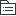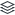# 公考言语理解与表达-选词填空

113
|
21

## 相关模板推荐

•## 选词填空 —— 作品大纲

•实词
• 考察内容
• 语境分析、实词匹配
• 常用词、常误用词
• 解题方法
• 词语辨析法
• 理性
• 词义轻重
• 词义侧重
• 色彩
• 感情
• 褒义
• 贬义
• 中性
• 态度倾向
• 语体
• 口语
• 书面
• 形象
• 事物行为状态颜色
• 语法
• 词性功能
• 名词
• 主宾
• 动词
• 形容词
• 定谓
• 词语搭配
• 固定
• 对应关系
• 表述功能
• 主被动
• 主客观
• 肯否定
• 语境分析法
• 逻辑关系
• 递进
• 正向照应，后比前重
• 转折
• 反向照应
• 因果
• 感情倾向一致，因果关系
• 并列
• 正向照应
• 反向照应
• 语义关系
• 解释说明
• 分句末尾，语意说明
• 归纳总结
• 句末，前文展开
• 修饰限定
• 句中，搭配和修饰限定
•成语填空
• 考查内容
• 意义相近，易混淆
• 易误用
• 解题方法
• 成语辨析
• 语义侧重点
• 成语中动词及修饰语
• 成语中不同的字
• 语义程度
• 语义轻重
• 感情色彩
• 褒义
• 贬义
• 中性
• 感情倾向
• 积极
• 消极
• 中性
• 语境分析
• 逻辑关系
• 正向、反向照应
• 递进转折因果并列选择
• 语义关系
• 提示
• 解释说明、归纳总结、修饰限定
• 字面照应
• 成语正确含义
• 题干含义
•虚词填空
• 概述
• 语法关系
• 副介连助叹拟声
• 解题方法
• 虚词辨析法
• 词性
• 虚词放在形容词和动词前
• 否定词必放“把”字前
• 功能
• 语法意义
• 易考:逻辑关系关键词
• 用法
• 语法意义相同，用法差异-语言经济性
• 语境分析法
• 递进
• 方向一致，后比前深
• 并列关系
• 同等重要
• 正向
• 反向
• 转折
• 方向相反，重点在后
• 让步
• 假设条件
• 假设
• 条件关系
• 必要条件
• 严谨
• 只有……才
• 充分条件
• 绝对化些
• 只要……就
• 选择因果……